Tip: Other languages are Google-Translated. You can visit the English version of this link.orRegisteror

## How to sum the absolute values in Excel?

Supposing you have a list of data which contains both positive numbers and negatives, and now you want to sum their absolute values which means all the negatives will be calculated as positives. For example, you want 10+(-10) would be 20 instead of 0. Are there any quick tricks to help you calculate the absolute values in Excel?

Sum the absolute values with formulas

### Easily sum the absolute values by Kutools for Excel's Formulas utility

Kutools for Excel collects many commonly-used formulas for Excel users to quickly apply complicate formulas without remember the formula exactly, such as the Sum absolute values formula, Add months to date formula, Add hours/minutes/seconds to time formula, Find the most common value formula, etc. Full Feature Free Trial 60-day!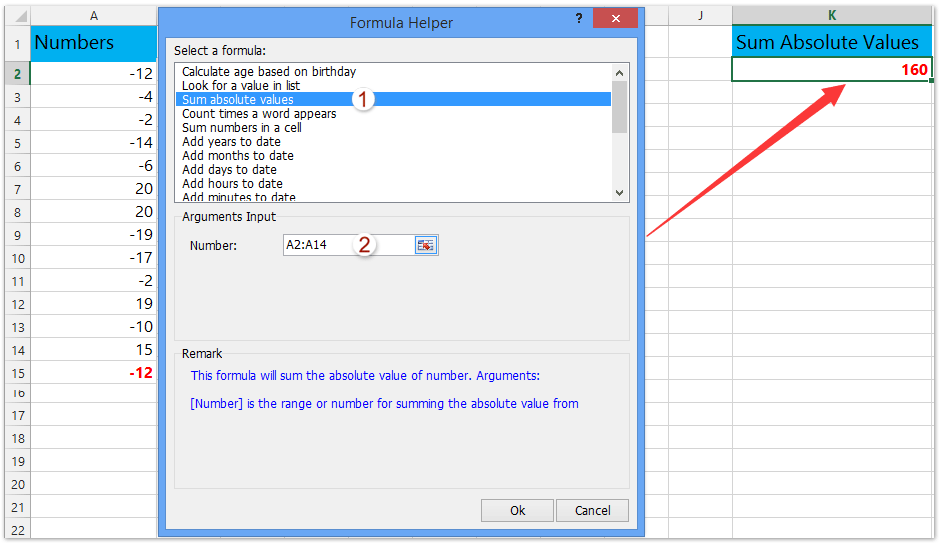####Sum the absolute values with formulas

Here are some cute formulas for you to sum the absolute values, please look at this:###### Save 50% of your time, and reduce thousands of mouse clicks for you every day!

Formula 1: SUMIF() function:

With the following two SUMIF functions, you can quickly calculate the sum of the absolute values, do as this:

1. In a blank cell which you want to put the result, enter this formula =SUMIF(A2:A11,">0") - SUMIF(A2:A11,"<0") or =SUM(SUMIF(A2:A11,{">0","<0"})*{1,-1}), and press the Enter key. See screenshots:Formula is too complicated to remember? Save the formula as an Auto Text entry for reusing with only one click in future! Read more…     Free trial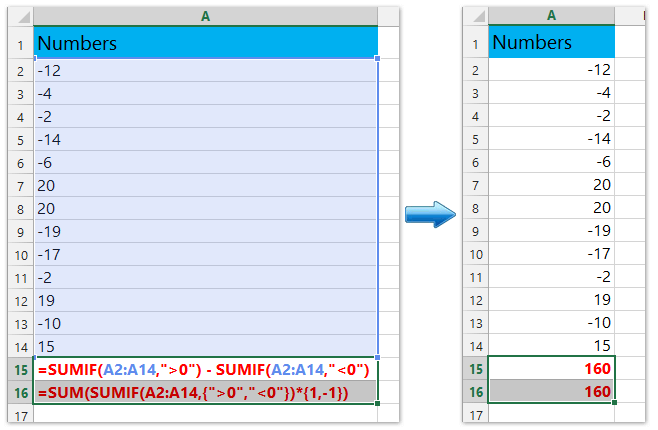Formula 2: SUMPRODUCT() function

This Sumproduct formula also can do a favor for you, please type the following formula: =SUMPRODUCT(ABS(A2:A11)), and press Enter key, then you will get the sum of the absolute values that you need.

Formula 3: SUM() Array Formula:

If you are interested in the array formula, I can talk about this Sum array formula. Please enter this: =SUM(ABS(A2:A11)) formula, and press Ctrl+Shift+Enter keys, which signifies this is an array formula, after pressing the keys, the result will be displayed and you'll see braces around the formula in the Formula bar: {=SUM(ABS(A2:A11))}.

####Sum the absolute values by changing all negative numbers to positive in Excel

Apart from formulas, we can change all negative numbers to positive ones and then sum up these numbers. And you can apply Kutools for Excel's Change Sign of Values utility to get it done with ease.

 Kutools for Excel - Combines more than 300 Advanced Functions and Tools for Microsoft Excel

1. Select a blank cell below the numbers whose absolute values you will add, type the formula =SUM(A2:A14) (A2:A14 is the number cells whose absolute values you will sum) into it, and press the Enter key. See screenshot: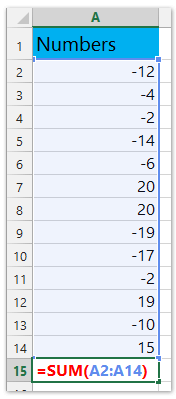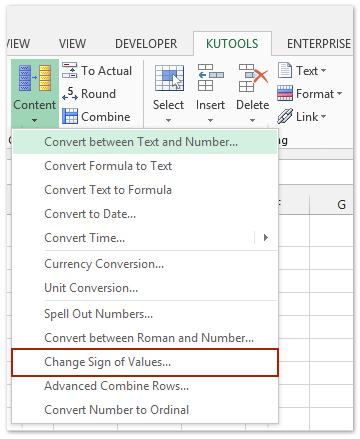2. Select these number cells (A2:A14 in our case), and click Kutools > Content > Change Sign of Values. See screenshot above.

3. In the opening Change Sign of Values dialog box, please check the Change all negative values to positive option, and click the Ok button.

And now all negative values are converted to positive values, and the summing result changes automatically. See screenshot below: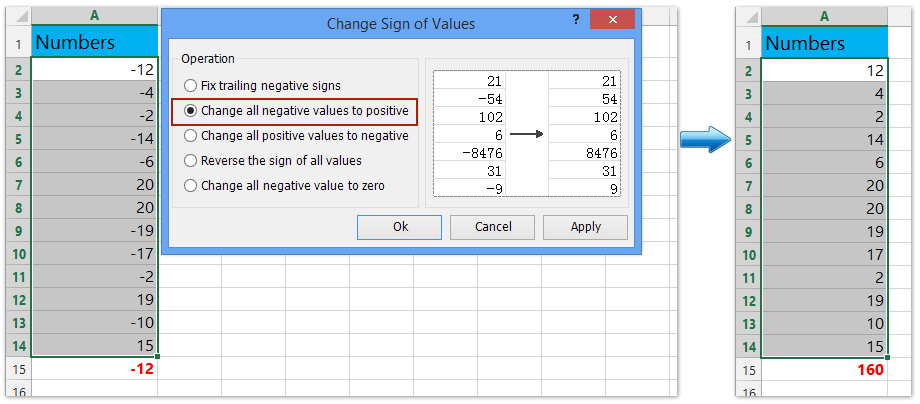Kutools for Excel - Includes more than 300 handy Excel tools. Full feature free trial 60-day, no credit card required! Get it now!

####Demo: sum the absolute values with Kutools for Excel

In this Video, the Kutools tab and the Kutools Plus tab are added by Kutools for Excel. If need it, please click here to have a 60-day free trial without limitation!

### Quickly extract numbers from text strings with Kutools function in Excel

Kutools for Excel's Kutools Functions utility provides an EXTRACTNUMBERS function to help you quickly extract numbers from mixed text strings with ease.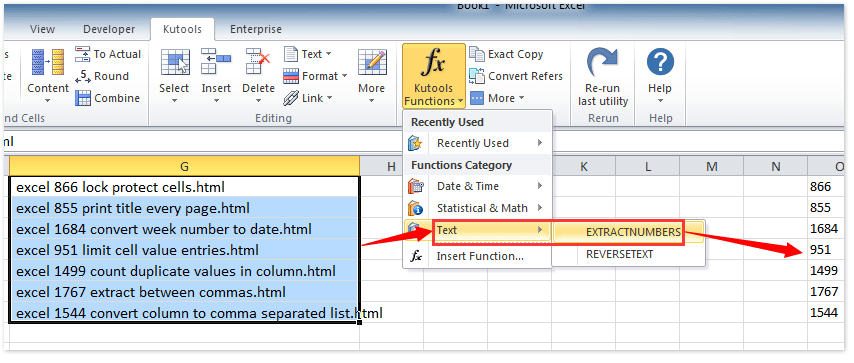Related articles:

How to sum only filtered or visible cells in Excel?

How to quickly sum every other or nth row/column in Excel?

### Kutools for Excel Helps You Always Finish Work Ahead of Time, and Stand Out From Crowd

• More than 300 powerful advanced features, designed for 1500 work scenarios, increasing productivity by 70%, give you more time to take care of family and enjoy life.
• No longer need memorizing formulas and VBA codes, give your brain a rest from now on.
• Become an Excel expert in 3 minutes, Complicated and repeated operations can be done in seconds,
• Reduce thousands of keyboard & mouse operations every day, say goodbye to occupational diseases now.
• 110,000 highly effective people and 300+ world-renowned companies' choice.
• 60-day full features free trial. 60-day money back guarantees. 2 years of free upgrade and support.

### Brings Tabbed Browsing and Editing to Microsoft Office, Far More Powerful Than The Browser's Tabs

• Office Tab is designed for Word, Excel, PowerPoint and Other Office Applications: Publisher, Access, Visio and Project.
• Open and create multiple documents in new tabs of the same window, rather than in new windows.
• Increases your productivity by 50%, and reduces hundreds of mouse clicks for you every day!Say something here...
symbols left.